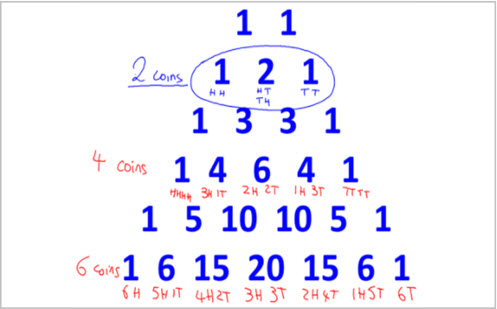Go to website

# Four coins

This inquiry lesson is aimed to extend and explore students' understanding of probability. Students flip four coins and are encouraged to demonstate their understanding through prompts: mathematical statements, equations or diagrams. Students will pose questions throughout the lesson based on their observations, which will help the teacher move between different levels of the lesson. Downloadable resources are provided to assist with teaching the topic.

Year level(s) Year 9
Audience Teacher
Teaching strategies and pedagogical approaches Collaborative learning, Differentiated teaching, Feedback, Questioning, Metacognitive strategies, Mathematics investigation
Keywords chance, probability, pascal's triangle, binomial expansion, probability trees

## Curriculum alignment

Curriculum connections Critical and creative thinking, English, Numeracy, Ethical understanding, ICT general capability
Strand and focus Number, Probability, Statistics, Apply understanding, Build understanding
Topics Chance and probability, Operating with number
AC: Mathematics (V9.0) content descriptions
AC9M9P01
List all outcomes for compound events both with and without replacement, using lists, tree diagrams, tables or arrays; assign probabilities to outcomes

Numeracy progression Understanding chance (P6)